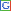welcome to Zahie.comMalaysia's leader said on Saturday communications aboard a missing jet were switched off and its course deliberately changed by someone on board before the aircraft disappeared a week ago, but stopped short of saying it had been hijacked. Ukraine braced on Saturday for a breakaway vote in Crimea as deadly violence flared again in the ex-Soviet country's tinderbox east amid the biggest east-west showdown since the Cold War. Finally, the TRS chief K Chandrashekhara Rao has broken his silence over possibility of an alliance with the Congress in Telangana. After saying a firm 'no' to merger with the Congress, KCR has now ruled out any alliance with the Congress in both Assembly and Lok Sabha elections to be held on April 30. The Bharatiya Janata Party's much anticipated list of Lok Sabha candidates is expected shortly which will end the the suspense over who will contest from Varanasi - its prime ministerial candidate Narendra Modi or party veteran Murli Manohar Joshi.The Delhi High Court has confirmed death penalty to all four convicts of the December 16 Delhi gangrape and murder case. The four were convicted in September 2013 for raping and murdering a 23-year-old paramedical student.Home About Us Archaeology Earth English Essays General Knowledge History India Social Studies Jokes Memoirs Pen Pals Quotations Riddles Science Sports Travel Trivia Wildlife Funny India Toons Short Story World Poem News Economics Biology Physics Links Contact Us
Home >> Physics >> Motion III

Motion III

Velocity/Time graph

Uniform Motion- The Velocity /Time graph of an object moving in uniform motion is a straight line which passes through the origin.

Slope = y2-y1/x2-x1                  Where y is taken from the distance and y2-y1

Where x is taken from the time and x2-x

Zero Acceleration- The graph of an object with zero acceleration is a straight line which is parallel to the x axis

Uniform Retardation- The graph of an object with uniform retardation is a straight line and the line meets the x axis when the object comes to rest.

Variable Retardation/Acceleration- The graph of an object with Variable Retardation/Acceleration is a curve line. The slope cannot be calculated.

Graphical Derivations of Equations of Motion

Graphical Derivation of First Equation

Consider an object moving with a uniform velocity u in a straight line. Let it be given a uniform acceleration a at time t = 0 when its initial velocity is u. As a result of the acceleration, its velocity increases to v (final velocity) in time t and S is the distance covered by the object in time t.

The figure shows the velocity-time graph of the motion of the object.

Slope of the v - t graph gives the acceleration of the moving object.

Thus, acceleration = slope = AB =

v - u = at

v = u + at 1st  equation of motion

Graphical Derivation of Second Equation

Distance travelled S = area of the trapezium ABDO

= area of rectangle ACDO + area of DABC

(v = u + at I equation of motion; v - u = at)

2nd Equation of motion

Graphical Derivation of Third Equation

S = area of the trapezium OABD.

Substituting the value of t in equation (1) we get,

2aS = (v + u) (v - u)

(v + u)(v - u) = 2aS [using the identity a2 - b2 = (a+b) (a-b)]

v2 - u2 = 2aS   3rd Equation of Motion

Circular Motion/Accelerated Motion

When an object moves along a circular path with constant speed its direction keeps on changing continuously hence it is known as acceleration motion. At any instant the direction of motion is along the tangent to the circle at that point.

 Spelling Beedifficulty level: easy hard expert score: - please wait...   spell the word: Spelling Bee provided by The Free Dictionary